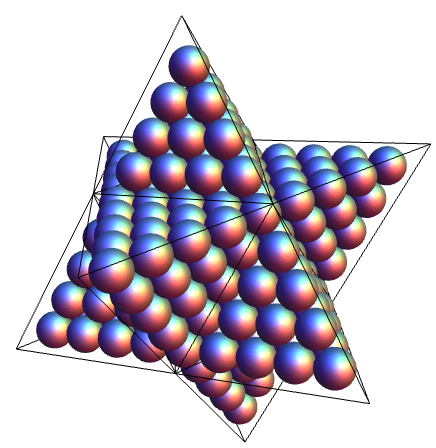# Stella octangula

In this rather popular video, Vi Hart experiments with the patterns that can be formed by connecting the vertices of a regular polygon with straight lines of equal length.

In addition to the obvious number-theoretic interpretation, there is a nice group-theoretic interpretation that generalises well to higher dimensions. For instance, Vi Hart’s ‘square star’ composed of four squares can be thought of as a 3-tuple, namely:

• The full group G = D32 of symmetries of the star;
• A normal subgroup H = D8 of symmetries fixing each of the components;
• The quotient group G/H = C4 of cyclic permutations.

With two dimensions, these things are really easy to find, and completely classified by Vi Hart’s video. On the other hand, the multidimensional analogues are really exceptional objects. The standard five-pointed pentagram has four three-dimensional versions, the Kepler-Poinsot polyhedra, and ten four-dimensional analogues, the Schläfli-Hess polychora. One of the Kepler-Poinsot polyhedra is the small stellated dodecahedron, immortalised in card by Joseph Myers:Of course, for the group theory to come into play non-trivially, we are interested in examples where we have a compound of polytopes, analogous to the six-pointed Star of David. Together with the restriction that both the convex hull and constituent polytopes are regular, the only possibilities are:

• Three 16-cells in four dimensions, with the same vertices as a 24-cell (quotient S3).
• Two tetrahedra in three dimensions, with the same vertices as a cube (quotient C2).

If the convex hull is not required to be regular, then other possibilities include two dual 24-cells in four dimensions, two dual simplices (in any number of dimensions), and six 16-cells in four dimensions (by combining two of the previous results).

Anyway, the unique three-dimensional example is the stella octangula, a compound of two dual tetrahedra. It appears twice in Escher’s Stars, and there’s even a variant of the Rubik’s cube based on it. As one can imagine, it has also been constructed by Joseph Myers:The visible surface can also be obtained by gluing eight regular tetrahedra to an octahedron, in the way that occurs in the dual tiling to the rhombic dodecahedral honeycomb.

### Stella octangula numbers

By analogy with triangular numbers, square numbers and tetrahedral numbers, the sequence of stella octangula numbers has been investigated. For instance, 245 is a stella octangula number, since we can fill a stella octangula with 245 spheres in a FCC arrangement:David Eppstein has even assembled 124 magnetic balls into a stella octangula, for the Wikipedia article on stella octangula numbers.

As with all three-dimensional figurate numbers, they are values of a cubic polynomial. Hence, if we try to find stella octangula numbers which are also square, we have the standard problem of locating integer points on an elliptic curve, of which there are finitely many. It was proved that the only such numbers are 1 (trivially) and 9653449.

A similar problem is to find pyramidal numbers which are square. The single non-trivial solution to this is much more well-known: 1² + 2² + … + 24² = 70². This is intimately related to a particular construction of the Leech lattice, where one begins with a much simpler (25+1)-dimensional even unimodular lattice in Minkowski space, and chooses the 24-dimensional plane of null vectors orthogonal to the null vector (70; 1, 2, 3, …, 24).

This entry was posted in Uncategorized. Bookmark the permalink.